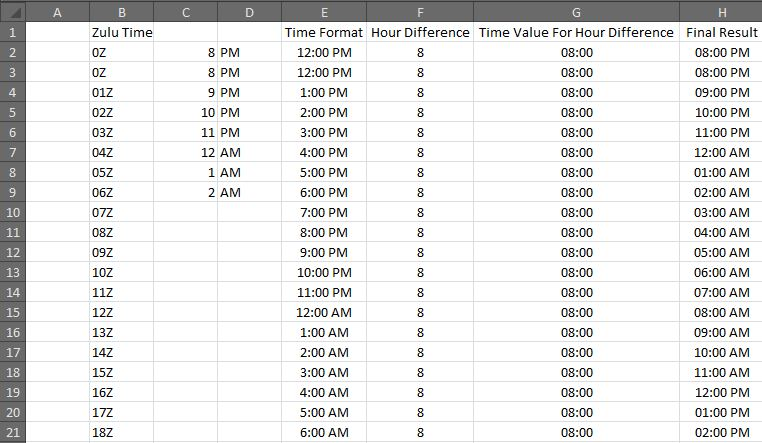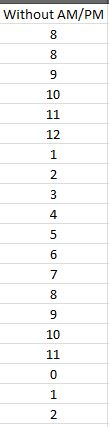# Converting time

Hello,

Trying to find a way to convert a list of time  values in Zulu to local time.  Column B has a list of values 01Z, 02Z, 03Z, etc... down to row 20.  I need in Column C and D to convert the time over to local time.  It would subtract the value from cell G1 from Zulu time and put the numeric digit in Column C and either PM or AM in Column D.  I have attached an example.
TIme_Convert.xlsxLast Comment
Jacques GedayShums FarukHi Sandra,

It won't be possible with just single formula.
First formula extracting numeric values from Col B and converting them into Time Format in E2
``````=TIME(TRUNC(ABS(LEFT(B2,1))),(ABS(LEFT(B2,1))+12 -TRUNC(ABS(LEFT(B2,1))))*60,0)
``````
Assuming your Hour Difference to GMT is +8, I have added another column F to have Hour Difference, again I will convert Hour difference to Time Format in G2 with below formula:
``````=TIME(TRUNC(ABS(F2)),(ABS(F2) -TRUNC(ABS(F2)))*60,0)
``````
Final result in H2 with below formula:
``````=IF(ISERROR(F2),"Unknown TimeZone", IF(F2<0,IF(E2<G2,E2-G2+2*TIME(12,0,0),E2-G2),E2+G2))
``````
Change the Col H format to hh:mm AM/PMIf you just want numeric values then use below formula, change Left function as per first two digits:
``````=IF(MOD(LEFT(B2,1)+8,24)=12,MOD(LEFT(B2,1)+8,24),MOD(LEFT(B2,1)+8,12))
``````Hope this helps...
I tweaked as per your requirement from here
Sandra_Zulu-Time_Convert.xlsxJacques GedayTHIS SOLUTION IS ONLY AVAILABLE TO MEMBERS.
View this solution by signing up for a free trial.
Members can start a 7-Day free trial and enjoy unlimited access to the platform.sandramac

Thank YouJacques GedaygowflowMicrosoft Excel

Microsoft Excel topics include formulas, formatting, VBA macros and user-defined functions, and everything else related to the spreadsheet user interface, including error messages.

144K
Questions
--
Followers
--
Top Experts
Get a personalized solution from industry experts

TRUSTED BY